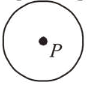# A perfectly diamagnetic sphere has a small spherical cavity at its centre,Question:

A perfectly diamagnetic sphere has a small spherical cavity at its centre, which is filled with a paramagnetic substance. The whole system is placed in a uniform magnetic field $\vec{B}$. Then the field inside the paramagnetic substance is :1. (1) $\vec{B}$

2. (2) zero

3. (3) much large than $|\vec{B}|$ and parallel to $\vec{B}$

4. (4) much large than $|\vec{B}|$ but opposite to $\vec{B}$

Correct Option: , 2

Solution:

(2) When magnetic field is applied to a diamagnetic substance, it produces magnetic field in opposite direction so net magnetic field inside the cavity of sphere will be zero. So, field inside the paramagnetic substance kept inside the cavity is zero.